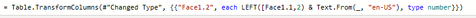cancel
Showing results for
Did you mean:Helper I

## Add prefix to column based on another columnI'm trying to break this data up into two columns that I can subtract from each other. So for example I'd like to change 1950/ 50 to 1950 - 1950 = 0

The problem is sometimes the number on the right of the / only has two digets. So for example 1940/60 should be 1940/1960.I first seperated the number into two columns based on the / using split column button.

Next I'd like to create some logic that says if there are less than 4 characters in Face1.2, append the left two characters from Face1.1 to Face1.2. I'm having trouble doing this. I tried using the prefix option to do this and changed the face to LEFT([Face1.1],2) but I got an error.Thanks for the help!

1 ACCEPTED SOLUTIONSuper User

HIi @left4pie2 , the following can be used in the Face.1.2 column in the transformcolumns function.

`Table.TransformColumns(#"Previous Step", [Face.1.2] , each if Text.Length(_) = 2 then "19" & _ else _ )`

In the next step, you can convert both text columns to Numbers.

2 REPLIES 2Super User

HIi @left4pie2 , the following can be used in the Face.1.2 column in the transformcolumns function.

`Table.TransformColumns(#"Previous Step", [Face.1.2] , each if Text.Length(_) = 2 then "19" & _ else _ )`

In the next step, you can convert both text columns to Numbers.Helper I

That worked, thanks!